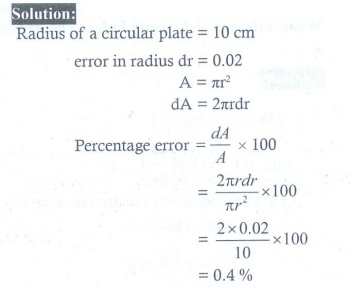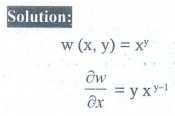Home | | Maths 12th Std | Exercise 8.8: Choose the correct answer

# Exercise 8.8: Choose the correct answer

Choose the correct or the most suitable answer from the given four alternatives :

EXERCISE 8.8

Choose the correct or the most suitable answer from the given four alternatives :

1. A circular template has a radius of 10 cm. The measurement of radius has an approximate error of 0.02 cm. Then the percentage error in calculating area of this template is

(1) 0.2%

(2) 0.4%

(3) 0.04%

(4) 0.08%2. The percentage error of fifth root of 31 is approximately how many times the percentage error in 31?

(1) 1/31

(2) 1/5

(3) 5

(4) 313. If u x, y ) = ex2+y2 , then Ōłéu/Ōłéx is equal to

(1) ex2+y2

(2) 2xu

(3) x2u

(4) y2u4. If v ( x, y ) = log(ex + ey ) , then Ōłév/Ōłéx + Ōłéy/Ōłév is equal to

(1) ex + ey

(2) 1 / ex + ey

(3) 2

(4) 15. If w( x, y ) = xy , x > 0 , then Ōłéw/Ōłéx is equal to

(1) xy log x

(2) y log x

(3) yxyŌłÆ1

(4) x log y6. If f ( x, y ) = exy, then Ōłé2f / ŌłéxŌłéy is equal to

(1) xyexy

(2) (1 + xy)exy

(3) (1 + y ) exy

(4) (1 + x ) exy7. If we measure the side of a cube to be 4 cm with an error of 0.1 cm, then the error in our calculation of the volume is

(1) 0.4 cu.cm

(2) 0.45 cu.cm

(3) 2 cu.cm

(4) 4.8 cu.cm8. The change in the surface area S = 6x2 of a cube when the edge length varies from x0 to x0 + dx is

(1) 12x0 + dx

(2) 12 x0 dx

(3) 6 x0 dx

(4) 6 xdx9. The approximate change in the volume V of a cube of side x metres caused by increasing the side by 1% is

(1) 0.3xdx m3

(2) 0.03x m3

(3) 0.03x2 m3

(4) 0.03x3 m310. If g ( x, y ) = 3x2 ŌłÆ 5 y + 2 y2 , x (t) = et and y (t) = cos t , then dg/dt is equal to

(1) 6e2t + 5sin t ŌłÆ 4 cos t sin t

(2) 6e 2t ŌłÆ 5sin t + 4 cos t sin t

(3) 3e2t + 5sin t + 4 cos t sin t

(4) 3e2t ŌłÆ 5sin t + 4 cos t sin t11. If f (x) = x / x+1 , then its differential is given by12. If u ( x, y ) = x2 + 3xy + y ŌłÆ 2019 , then Ōłéu/Ōłéx|(4,ŌłÆ5) is equal to

(1) ŌłÆ4

(2) ŌłÆ3

(3) ŌłÆ7

(4) 1313. Linear approximation for g ( x) = cos x at x = ŽĆ/2 is

(1) x + ŽĆ/2

(2) ŌłÆ x + ŽĆ/2

(3) x ŌĆō ŽĆ/2

(4) ŌłÆ x ŌĆō ŽĆ/214. If wx, y , z= x2 ( y ŌłÆ z ) + y2 ( z ŌłÆ x) + z2 ( x ŌłÆ y) , then Ōłéw/Ōłéx + Ōłéw/Ōłéy + Ōłéw/Ōłéz is

(1) xy + yz + zx

(2) ( y + z)

(3) ( z + x)

(4) 015. If f x, y , z) = xy + yz + zx , then fx ŌłÆ fz is equal to

(1) z ŌłÆ x

(2) y ŌłÆ z

(3) x ŌłÆ z

(4) y ŌłÆ xTags : Differentials and Partial Derivatives | Mathematics , 12th Maths : UNIT 8 : Differentials and Partial Derivatives
Study Material, Lecturing Notes, Assignment, Reference, Wiki description explanation, brief detail
12th Maths : UNIT 8 : Differentials and Partial Derivatives : Exercise 8.8: Choose the correct answer | Differentials and Partial Derivatives | Mathematics